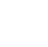# Java Decision Making And Branching

“Decision making and branching” is one of the most important concepts of computer programming. Programs should be able to make logical (true/false) decisions based on the condition provided. So controlling the execution of statements based on certain condition or decision is called decision making and branching.

## 1.If Statements

The if statement is used to test the condition. It checks boolean conditions true or false. There are various types of if statements in Java.

• Simple if statement
• if-else statement
• nested if statement

### i) Simple if Statement :

The Java if statement tests the condition. It executes the *if block* if the condition is true. Where boolean_expression is a condition.

Syntax:

if(Boolean_expression) {
// Statements will execute if the Boolean expression is true
}
Statement-X;

Example:

public class Branching {

public static void main(String args[]) {
int num = 10;

if( num < 20 ) {
System.out.print("Welcome to ShapeAI");
}
}
}
/*EXPLANATION:
hence num value 10 is less than 20, it executes the statement inside if block*/

/*OUTPUT:
Welcome to ShapeAI */

### ii) if-else Statement :

The Java if-else statement also tests the condition. It executes the if block, if the condition is true otherwise else block, is executed.

Syntax:

if(Boolean_expression) {
// Executes when the Boolean expression is true
}else {
// Executes when the Boolean expression is false
}
Statement-X;

Example:

public class Branching {

public static void main(String args[]) {
int num = 30;

if( num < 20 ) {
System.out.print("This is if statement");
}else {
System.out.print("This is else statement");
}
}
}
/*EXPLANATION:
executes the else statement hence the num value 30 is greater than 20 */

/*OUTPUT
This is else statement */

### iii) if-else-if ladder Statement :

The if-else-if ladder statement executes one condition from multiple statements.

Syntax:

if(Boolean_expression 1) {
// Executes when the Boolean expression 1 is true
}else if(Boolean_expression 2) {
// Executes when the Boolean expression 2 is true
}else if(Boolean_expression 3) {
// Executes when the Boolean expression 3 is true
}else {
// Executes when the none of the above condition is true.
}
Statement-X;

Example:

public class Branching{

public static void main(String args[]) {
int num = 30;

if (num == 10) {
System.out.print("Value of num is 10");
} else if (num == 20) {
System.out.print("Value of num is 20");
} else if (num == 30) {
System.out.print("Value of num is 30");
} else {
System.out.print("This is else statement");
}
}
}

/*EXPLANATION:
executes the second else if block hence the num value 30 is equal to 30 */

/* OUTPUT
Value of X is 30 */

### iv) nested if statement :

The nested if statement represents the if block within another if block. Here, the inner if block condition executes only when the outer if block condition is

Syntax:

switch(expression) {
case value :
// Statements
break;

case value :
// Statements
break;

// You can have any number of case statements.
default : // Optional
// Statements
}
Statement-X;

Example:

public class Switching {

public static void main(String args[]) {

case 'A' :
System.out.println("Excellent!");
break;
case 'B' :
System.out.println("Good");
break;
case 'C' :
System.out.println("Well done");
break;
default :
}
}
}

/*EXPLANATION:
The given expression grade is assigned with 'C'. hence it matches with the case 'C'.
So case 'C' statement "Well done" is executed*/

/*OUTPUT:

Well done